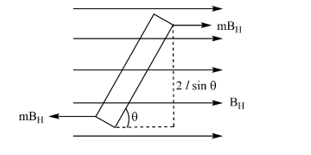Magnetic dipole in a uniform magnetic field
Question

# The restoring torque for a magnet oscillating in the vibration magnetometer is provided by

Easy
Solution

##$\tau =\left(m{B}_{H}\right)2l\mathrm{sin}\theta \tau$$=\left(m.2l\right){B}_{H}\mathrm{sin}\theta$$⇒\tau =\mu {B}_{H}sin\theta$

Get Instant Solutions